﻿ 基于模版匹配的阵列特征高精度定位算法 High-Precision Positioning Algorithm for Array Features Based on Template Matching

Artificial Intelligence and Robotics Research
Vol. 08  No. 04 ( 2019 ), Article ID: 32617 , 7 pages
10.12677/AIRR.2019.84021

High-Precision Positioning Algorithm for Array Features Based on Template Matching

Weiren Xiao

School of Electrical Engineering and Automation, Tianjin Polytechnic University, Tianjin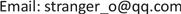Received: Oct. 8th, 2019; accepted: Oct. 16th, 2019; published: Oct. 23rd, 2019ABSTRACT

In combination with the actual medical test paper production, there is a phenomenon where the quality inspection link relies on manual visual inspection; the efficiency is low; the misjudgment rate increases with the working time, and there is also a health risk to the production workers. This article goes from hardware equipment to software algorithms. A set of automatic inspection system for test strip based on machine vision was designed. The traditional template matching algorithm was improved. The template matching was divided into two levels to make the matching search in the X and Y dimensions. The calculation method is optimized due to the excessive side effects of calculation, so that the resolution is high (0.04 mm) at the same time in a large field of view (310 * 330 mm), and the efficiency is still good, so that it does not increase. The time of the quality inspection process of the product significantly reduces the misjudgment rate.

Keywords:Machine Vision, Template Matching, High Precision1. 引言

2. 总体方案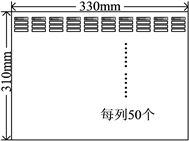Figure 1. Medical test paper overall overviewFigure 2. Test strip unit and drop area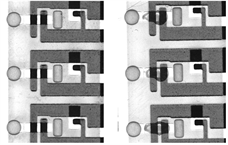Figure 3. Comparison between drop test paper and non-drip test paper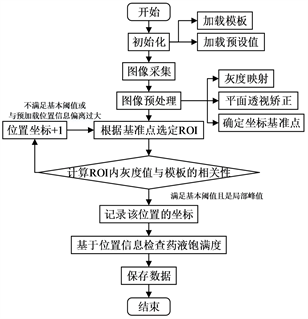Figure 4. Algorithm block diagram

3. 图像预处理部分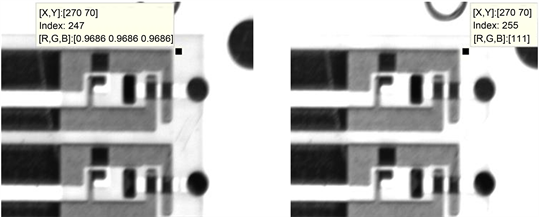Figure 5. Comparison before and after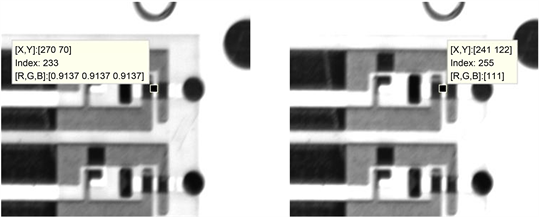Figure 6. Comparison before and after pretreatment of the drop area

4. 定位算法

1) 首先，选定模板，它必须能区分每个阵列单元，拥有的特征需要与单元内有较高相关性而与单元外(单元间隙)有较低的相关性。

2) 计算模板与待匹配子图的相关性或差异性，通过在某一位置的邻域内寻找相关性最大或差异性最小的子图。

3) 遍历搜索整张图片后得到所有子图最佳的定位点。

$D\left(i,j\right)={\sum }_{m=1}^{M}{\sum }_{N=1}^{N}{\left[Sij\left(m,n\right)-T\left(m,n\right)\right]}^{2}$

$cc\left(i,j\right)=\frac{{\sum }_{m=1}^{M}{\sum }_{n=1}^{N}Sij\left(m,n\right)×T\left(m,n\right)}{\sqrt{{\sum }_{m=1}^{M}{\sum }_{N=1}^{N}{\left[Sij\left(m,n\right)\right]}^{2}}\sqrt{{\sum }_{m=1}^{M}{\sum }_{N=1}^{N}{\left[T\left(m,n\right)\right]}^{2}}}$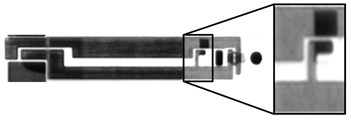Figure 7. Selected template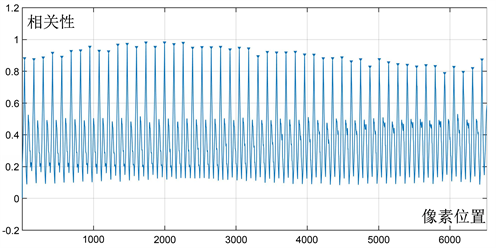Figure 8. Relationship between position and cc value in the Y direction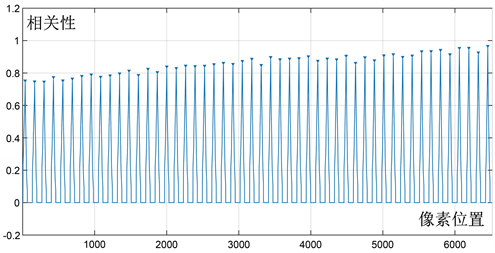Figure 9. Relationship between the jump positioning position and the cc value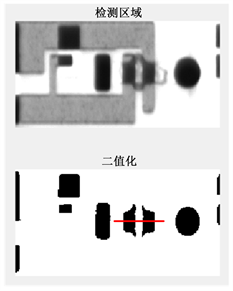Figure 10. ROI area and template preciselypositioned along the X direction

5. 结论

High-Precision Positioning Algorithm for Array Features Based on Template Matching[J]. 人工智能与机器人研究, 2019, 08(04): 190-196. https://doi.org/10.12677/AIRR.2019.84021

1. 1. Wu, X.Y., Xu, W.D., Li, L.H., et al. (2011) An Interactive Segmentation Method Using Graph Cuts of Mammographic Masses. In-ternational Conference on Bioinformatics & Biomedical Engineering, Wuhan, 10-12 May 2011, 1-4.
https://doi.org/10.1109/icbbe.2011.5780190

2. 2. 李彬, 罗彪. 机器视觉在物体位姿检测中的应用[J]. 传感器与微系统, 2016, 35(2): 150 -153.

3. 3. 杨剑. 大尺寸视觉测量精度的理论和实验研究[D]: [博士学位论文]. 北京邮电大学, 2010.

4. 4. 王道威. 基于OpenCV的图像匹配算法及其靶标定位应用[D]: [硕士学位论文]. 华中科技大学, 2016.

5. 5. 党晓军, 尹俊文. 一种基于模板匹配的运动目标跟踪方法[J]. 计算机工程与应用, 2010, 46(5): 173-176.

6. 6. 邵文坤, 黄爱民, 韦庆. 动态场景下的运动目标跟踪方法研究[J]. 计算机仿真, 2006(5): 181-184.

7. 7. 张彦梅, 宋扬. 模板匹配方法在高速目标跟踪中的应用[J]. 北京理工大学学报, 2006(11): 1026-1029.

8. 8. 邹承明, 薛栋, 郭双双, 赵广辉. 一种改进的图像相似度算法[J]. 计算机科学, 2016, 43(6): 72-76.

9. 9. Vock, R., Dieckmann, A., Ochmann, S. and Klein, R. (2019) Fast Template Matching and Pose Estimation in 3D Point Clouds. Computers & Graphics, 79, 36-45.
https://doi.org/10.1016/j.cag.2018.12.007

10. 10. 盛敏, 刘双庆, 王婕, 苏本跃. 基于改进模板匹配的智能下肢假肢运动意图实时识别[J/OL]. 控制与决策, 2019, 1-10 [2019-10-12].
https://doi.org/10.13195/j.kzyjc.2019.0070.

11. 11. 荆鑫. 基于模板匹配的视觉分拣方法及应用研究[D]: [博士学位论文]. 哈尔滨工业大学, 2017.